# OECD Nutrient balance data analysis 6 - making a panel data using R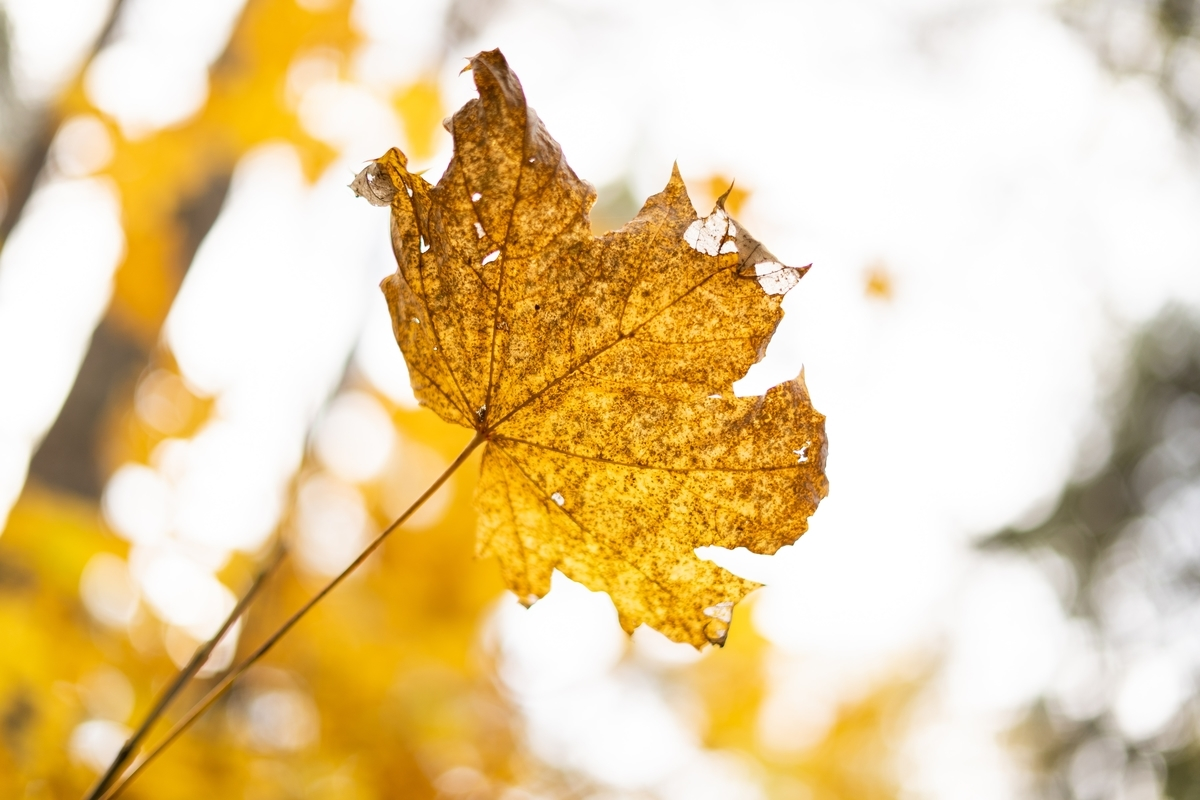Photo by Philip Myrtorp on Unsplash

This post is following of above post.

Since I made several objects, let me confirm what objects there is.

ls() function shows current object list.

So far, I have df_raw, df1, df2 and df3 for data frame.

gimpse() function is useful for what variables a data frame has.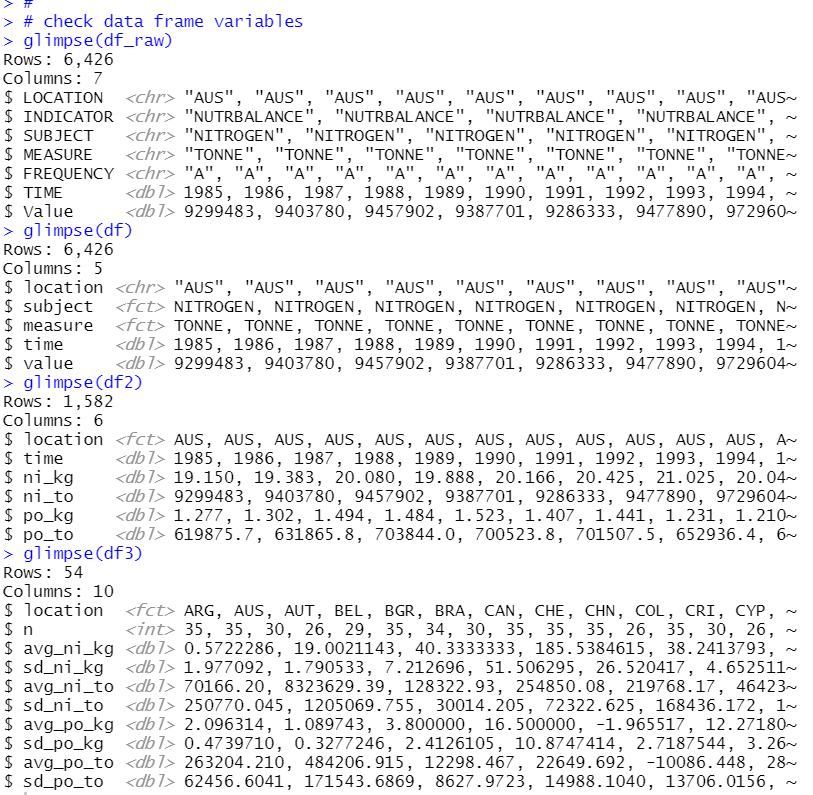df2 is like apanel data, so I will make panel data frame with plm packages's pdata.frame() function.

Firstly, I check how many observations each time has.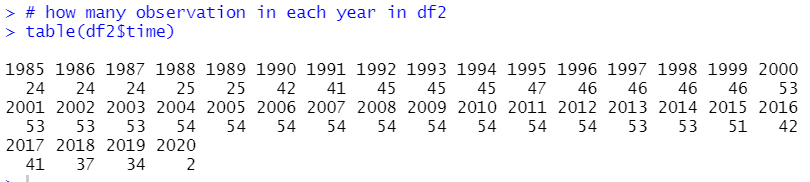From 2004 to 2012, there are 54 observations. So, I will use only from 2004 to 2012 data to make a panel data.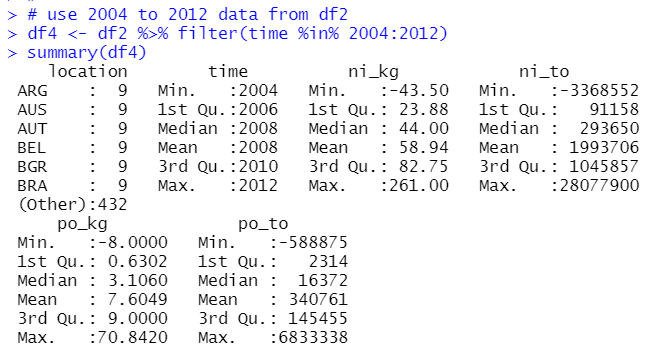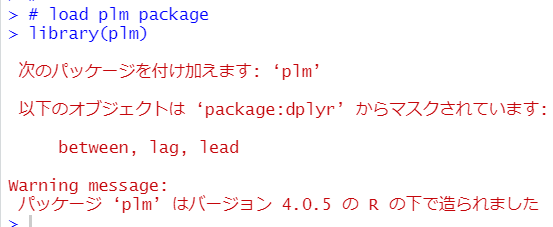Next, I use pdata.frame to make a panel data frame from df4.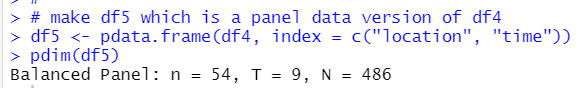All right. df 4 and df5 have the same data, but df4 is simple data frame while df5 is a panel data.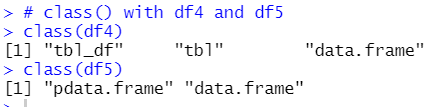I want to do regression analysis with df4 or df5 data, so I will change location and time to factor type.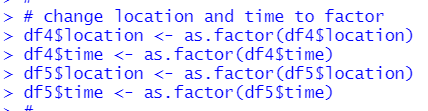Let's see summary for df4 and df5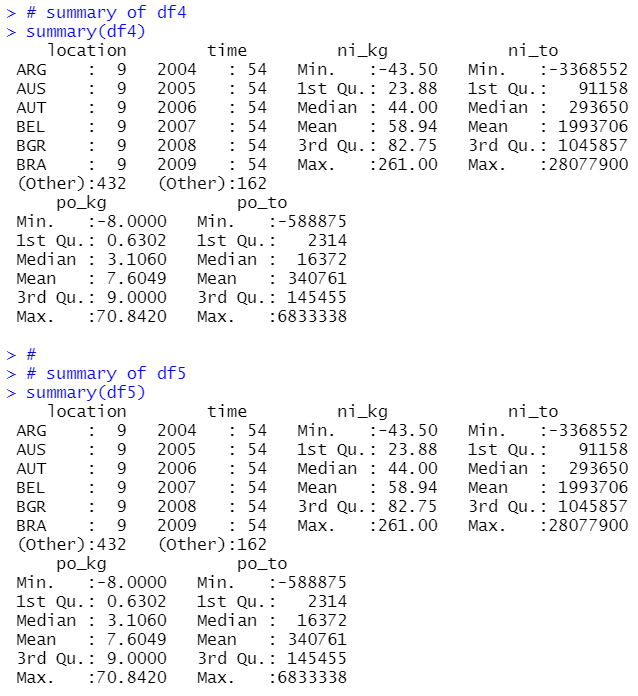Since ni_kg and ni_to, po_kg and po_to are very different value leve, I make scaled variables with scale() function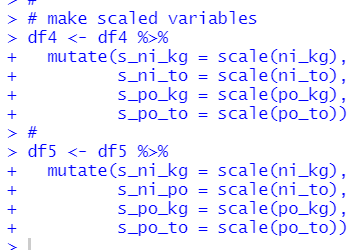Again, let's see summary of df4 and df5.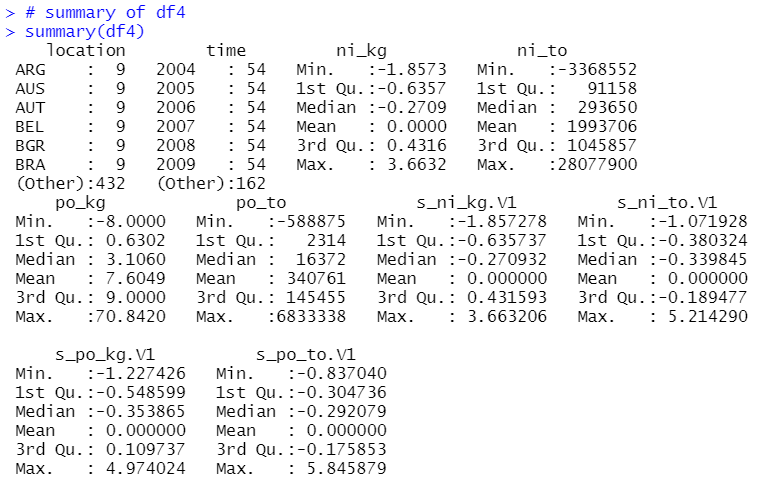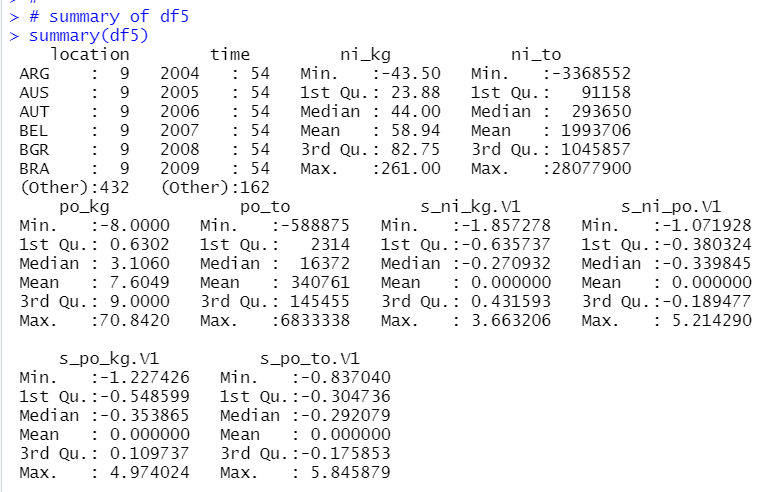Scaled variables are mean 0 and standard deviation 1.

That's it. Thank you!

The next post is

To read from the 1st post,